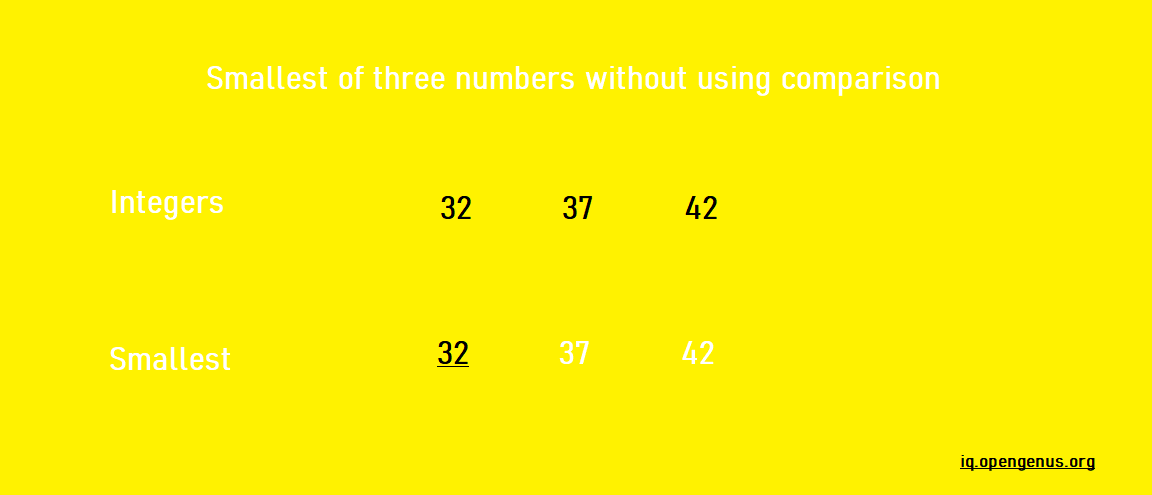×

Search anything:

# Find smallest of 3 integers without comparison

#### Algorithms bitwise algorithm List of Mathematical AlgorithmsGet this book -> Problems on Array: For Interviews and Competitive Programming

You must be mindful of what you're writing in your code while building any program. That way, even if you are given a simple problem, you may add your answer to make it appear more inventive. This not only improves your problem-solving abilities, but it also boosts your creativity as a coder. It may seem challenging at first, but as you continue to answer different problems, you'll soon be able to add your own unique response that distinguishes you from others.

As a result, we're going to do the same today. We'll approach the comparison problem in a different way.

In this guide, we will go through how to get the smallest of three numbers without using Comparison operation. We have presented 3 different techniques using decrement, division and shift operation.Without using any comparison operators, how do you obtain the minimum of three integers? There are several techniques for doing so, some of which are listed below.

1. Make use of decrement
2. Using division operator
3. Using subtraction and shift

# Make use of decrement

Though we can easily find the smallest number, however, the constraint is we cannot use any comparison operators. Therefore we will use subtraction to find the smallest integer.

This method uses the concept that the smallest number is the one that is closest to zero.

We will first create a variable i and set its value to 0. Decrement 1 from a, b, and c in a loop, then increase i each time. The smallest is the number that becomes 0 first. i will hold the minimum of 3 once the loop has ended.

``````int minimum(int a, int b, int c)
{
int i = 0;
while (a != 0 && b != 0 && c != 0) {
a--;  b--; c--;
i++;
}
return i;
}
``````

To better understand this, let's see an example. Suppose a = 3, b = 5 and c = 2. Let the counter variable be initialized as zero, i.e, i = 0.

Iteration 1:
a-- = 3-1 = 2,
b-- = 5-1 = 4,
c-- = 2-1 = 1,
i++ = 1

Iteration 2:
a-- = 2-1 = 1,
b-- = 4-1 = 3,
c-- = 1-1 = 0,
i++ = 2

Since, c = 0 and i = 2, the smallest of 3, 5 and 2 is 2.

Note: The drawback of this method is that it cannot be extended to find the smallest for any input < 0.

However, the time taken by this code is O(n), where n is the smallest. If we use the comparison operator, it takes constant time O(1).

The constant time method for finding the minimum of three numbers is shown below.

# Using division operator

We know that if the denominator is greater than the numerator, integer division will result in zero, i.e a / b = 0 (if a < b).

``````int minimumOfTwo(int a, int b)
{
if(a/b != 0)
return b;
else
return a;
}
int minimumOfThree(int a, int b, int c)
{
return minimumOfTwo(a, minimumOfTwo(b, c));
}
``````

We'll now look at an example of the division technique in action. Here, a = 78, b = 88 and c = 68.

int(b/c) = int(88/68) = 1. Since b/c != 0, therefore c < b.

int(a/c) = int(78/68) = 1. Since a/b != 0, therefore c < a.

Hence, the minimum of a(78), b(88) and c(68) is c(68).

Note: Like the above method, this method fails for any input < 0.

# Using subtraction and shift

``````int minimumOfTwo(int a, int b){
return  b + ((a - b) & ((a - b) >>
(sizeof(int) * CHAR_BIT - 1)));
}

int minimumOfThree(int a, int b, int c)
{
return min(a, min(b, c));
}
``````

This approach changes the difference of a and b by 31 (Assuming that size of int = 4 bytes).

• If (a - b) < 0, then (a - b) >> 31 will be 1.
• If (a - b) >= 0, then (a - b) >> 31 will be 0.

Therefore, if a >= b, the minimum is a + (a-b) & 0, which equals b.

Example : Let a = 12, b = 15 and c = 5.

b-c = 15-5 = 10 which is greater than 0. So, (b-c) >> 31 = 0.
Minimum of b and c = c + (b-c) & 0 = 5 + (15-5) & 0 = 5 which is c.

a-c = 12-5 = 7 which is greater than 0. So, (a-c) >> 31 = 0.
Minimum of a and c = c + (a-c) & 0 = 5 + (12-5) & 0 = 5 which is c.

Hence, the smallest of a(12), b(15) and c(5) is c(5).

Note: This method works for both positive as well as negative integers.

These were some techniques for determining the smallest of three integers without comparing them.

Now let's test our knowledge.

Question: What is the time complexity of finding the smallest of three numbers using repeated subtraction?

With this article at OpenGenus, you must have the complete idea of how to Find smallest of 3 integers without comparison.#### Harshita Singh

Harshita Singh is pursuing her B. Tech in CSE from Harcourt Butler Technical University, Kanpur (2023). She is an Intern at OpenGenus.

Find smallest of 3 integers without comparison